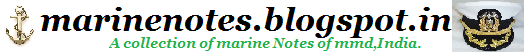Home » » LONGITUDINAL WEIGHT SHIFTS

# LONGITUDINAL WEIGHT SHIFTS

LONGITUDINAL WEIGHT SHIFTS

When a weight is shifted longitudinally (fore 'n' aft) the net effect on a ship is similar to a see-saw, one end goes up and the other goes down. The pivot of the "see-saw" is located at the Longitudinal Center of Flotation (LCF).To calculate the effect of shifting a weight longitudinally on the ships drafts, follow these steps:
1. Calculate the Trimming Moment (TM):2. Calculate the Change in Trim (CT):3. Calculate the change in forward draft (DdFWD):The + or - sign depends on the location of LCF. If LCF is aft of MP use "+" and if LCF is forward of MP use "-".
4. Calculate the change in aft draft (DdAFT):NOTE: If the weight was shifted forward, DdFWD will be positive and DdAFT will be negative. If the weight was shifted aft,DdAFT will be positive and DdFWD will be negative.
Example Problem
The FOWK just completed a transfer of 6500 gallons of diesel fuel (diesel = 322 Gallons/LT). The fuel is now located 135 FT forward of it's original position. Prior to transfer, the ships drafts were 16'9" fwd and 17'3" aft. Design Drag is 0.
LBP is 450 FT, MT1"=825 FT-Tons/inch and LCF is 24 FT aft of MP. The CHENG wants to know the new drafts and trim.
1. Calculate weight of fuel transferred:2. Calculate the trimming moment:3. Calculate the change in trim:4. Calculate the change in forward draft:5. Calculate the change in aft draft:NOTE: Since the weight was shifted forward, the draft change at the bow is positive, and at the stern is negative.

6. Determine the new drafts and trim:
Forward Aft Trim
Original: 16'9.00" 17'3.00" 6.0" b/s
Change: + 1.83" - 1.47" 3.3" b/b
Final: 16'10.83" 17'1.53" 2.7" b/s